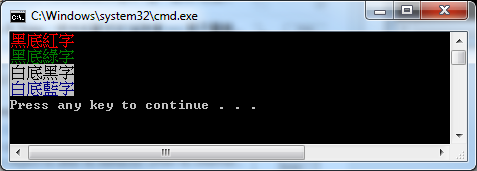``````call :AppendColorText [TextColor] [DisplayText]
call :AppendColorTextLine [TextColor] [DisplayText]
``````

``````0 = 黑色
1 = 藍色
2 = 綠色
3 = 藍綠色
4 = 紅色
5 = 紫色
6 = 黃色
7 = 白色
8 = 灰色
9 = 淡藍色
A = 淡綠色
B = 淡藍綠色
C = 淡紅色
D = 淡紫色
E = 淡黃色
F = 亮白色
``````

``````call :AppendColorTextLine 0C "黑底紅字"
``````